# Using the Locked Rotor Test to find parameters of DC motor?

• Engineering

## Homework Statement

A locked rotor test is performed on a permanent magnet DC machine with the following results:
Terminal Voltage 5 V DC
Input Current 10 A DC
Locked rotor torque. 20 Nm
a) Making suitable approximations deduce the effective rotor resistance in ohms?
b) Making suitable approximations deduce the torque constant kφ in Nm/A?
c) If a light running test was now performed with a terminal voltage of 50V DC what approximate shaft speed in rpm would you expect?
I did some calculations, however I am not sure if what I am doing is correct.

## Homework Equations

[/B]
Short circuit current at normal voltage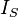is the short circuit current at voltage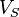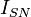is the short circuit current at normal voltage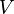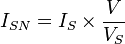Short circuit power factor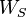is the total input power on short circuit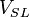is the line voltage on short circuit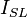is the line current on short circuit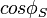is the short circuit power factor
Leakage reactance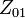is the short circuit impedance as referred to stator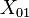is the leakage reactance per phase as referred to stator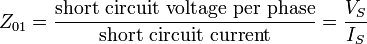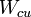is the total copper loss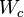is the core loss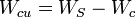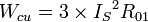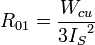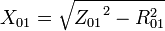## The Attempt at a Solution

[/B]
a) V/I= R => 0.5 ohms
b)Torque = k*Ia => Torque/Ia = k = 20 N*m/10 = k = 2
c) w= k*v => 50v*2= 100 rpm

I am not too sure if these are correct, it would be great if someone can help! Thank you :)

Last edited by a moderator:

It looks like you've copied in some equations from a Wikipedia page, but note that it deals with an induction machine, not a PM DC machine.

Your answers look correct to me. You could perhaps qualify them a bit by using your motor model, which I guess, in steady state, is given by:
$$V = RI_a + k\omega$$
What is it you're not sure about?

Edit:
I missed the (c) part, which you have the wrong answer for. You should consider what the back-EMF should be for it to balance the terminal voltage of 50 V.

Last edited:
NascentOxygen
Staff Emeritus
Equations that involve power factor and leakage reactance per phase are for AC motors.

Your answer to (b) wil be correct when you give it units.
I think you have insufficient information to be able to answer part (c).

I think you have insufficient information to be able to answer part (c).
I assume 'light running test' is equivalent to 'no-load test', so it'll accelerate until the terminal voltage is balanced by its back-EMF, but otherwise, I'd agree.

Thanks guys! I was confused because I searched through my textbook and it said that the no-load test was only for the AC machines and didn't mention anything about the DC. Hence, I used those equations I found on Wikipedia. I assumed that for a locked rotor test, the w would be 0. so V=RIa and that would make a=0.5 ohms and then for C I have no idea how to approach it. Even with a back-emf (how would I even calculate that? haha) Thank you!

so V=RIa and that would make a=0.5 ohms and then for C I have no idea how to approach it.
The motor will accelerate until its terminal voltage equals the back-EMF it's generating, because at that point there isn't any voltage to drive current into the armature, i.e. there's no torque to continue acceleration.

If the back-EMF is equal to ##k\omega##, what does that tell you the angular velocity ##\omega## is in steady state with a terminal voltage of 50 V?

NascentOxygen
Staff Emeritus